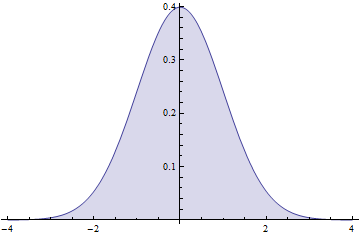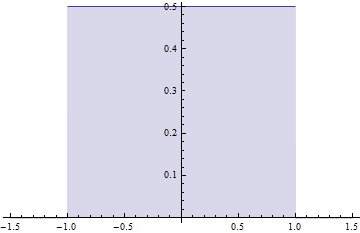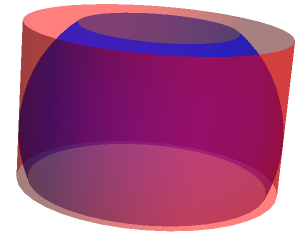# A slice of π

If I were more diligent and could actually be bothered to tag and categorise my blog posts, this one would belong in the esoteric realm of ‘probabilistic geometry’.

Suppose we have three variables, X, Y and Z, which are independent and random, determined by the bog-standard normal distribution with mean 0 and variance 1. The probability distribution function of each variable looks something like this:I encountered a puzzle somewhere, where you have to obtain a uniform random distribution just by applying basic operations to these three variables. A uniform distribution looks like this:How, then, is it possible to convert something as smooth and curvaceous as the normal distribution into a discrete and discontinuous uniform distribution? Surely it’s impossible? You may want to have a go at it yourself before I reveal all.

Somewhat surprisingly, we can indeed solve the puzzle by using a theorem of Archimedes. When a sphere is inscribed in a cylinder, and an arbitrary horizontal ‘slice’ is taken, the curved surface areas of the sphere and cylinder are identical:The Archimedean map projection involves projecting the surface of the Earth (assumed to be a sphere) onto a circumscribing cylinder. By Archimedes’ theorem, areas are preserved in this matter. The sphere can then be unrolled out to form a flat map. Usually, however, people use the Mercator projection instead, which preserves angles instead of areas. You may recognise angle-preserving (conformal) maps from Escher’s Circle Limit pictures.

Returning to the original problem, we can view each of X, Y and Z as axes in three-dimensional space and obtain a three-dimensional probability density function. This is the product of the probability density functions of the three original variables:Since it depends only on X^2+Y^2+Z^2, the squared distance from the origin, it must therefore have spherical symmetry. We can thus obtain a uniform distribution over the surface of the unit sphere by ‘normalising’ X, Y and Z to ensure that the squared distance from the origin is 1.If we let the x-axis be the axis of the cylinder, applying the Archimedean projection will not affect the value of X’. Since all slices of a cylinder are identical, X’ must therefore be uniformly distributed over the interval [-1,1]. Success!

I’ll end on another puzzle, introduced to me by Geoff Smith. I can’t recall the exact wording of the original statement of the problem, so I’ll improvise:

Deep within the Arctic Circle, a group of elves were busy making mathematical puzzles to deliver to children on Christmas Day. Unfortunately, there was a hole in the Arctic ice of diameter one metre, and they were worried that one of Geoff’s reindeer may fall into the tundra and perish. Hence, in the interests of ‘elf and safety*, it had to be covered with wooden planks. Obviously, we can use parallel planks (with a combined width of one metre) to cover it. Is it possible to have a combined width less than one metre if we adopt an intricate criss-crossing structure instead?

* Pun-believable joke conceived by Andrew Carlotti.

This entry was posted in Uncategorized. Bookmark the permalink.